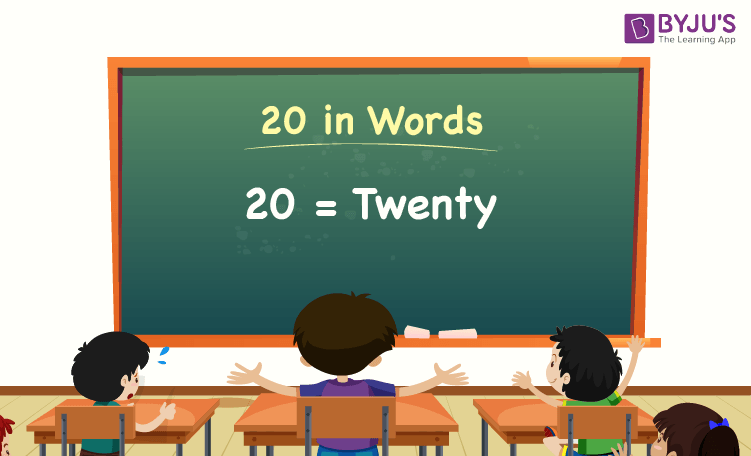# 20 in Words

20 in words is Twenty. The number name of 20 can be written using the ones, tens, hundreds, thousands place of a number. Thus, the place value chart helps us to write the number 20 in words. For example, if you earned Rs. 20 in a day, you can write, “I have earned Rs. Twenty in a day”.

 20 in Words: Twenty

In this article, let us learn the spelling of 20 in words, and look at the solved examples in detail.

## How to Write 20 in Words?As discussed above, the number 20 in words can be written using the place value. Thus, for any number, first, we have to identify the place value of each digit of the given number. Hence, for the number 20, the digit in one’s place is 0, and the digit in ten’s place is 2.

1’s place of 20 is 0.

10’s place of 20 is 2.

Since the number 20 is a two-digit number, there are no hundreds and thousands.

Thus, the place value chart for 20 is:

 Tens Ones 2 0

Therefore, the number 20 in words is twenty.

### Examples

Example 1:

Find the value of 120 – 100. Describe the value in words.

Solution:

Given expression: 120 – 100

⇒ 120 – 100 = 20

So, the value of 120 – 100 is 20.

Hence, 20 in words is twenty.

Example 2:

Express the value of twenty plus forty in words.

Solution:

Twenty = 20

Forty = 40

Twenty plus forty = 20 + 40 = 60

Hence, the value of twenty plus forty is sixty in words.

## Frequently Asked Questions on 20 in Words

Q1

### Write 20 in words.

20 in words is twenty.

Q2

### Express the value of 40 – 20 in words.

Simplifying 40 – 20, we get 20. Hence, 20 in words is twenty.

Q3

### How to write twenty in numbers?

Twenty in numbers is 20.

Q4

### Is the number 20 an odd number?

No, the number 20 is not an odd number.

Q5

### Is 20 a composite number?

Yes, 20 is a composite number.